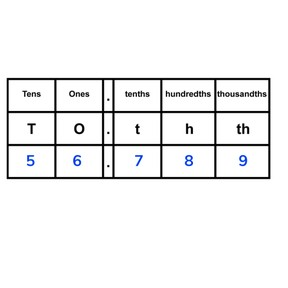Place value- know the value of the decimal place

# Place value- know the value of the decimal place

Place value- know the value of the decimal place

No account needed.8,000 schools use Gynzy92,000 teachers use Gynzy1,600,000 students use Gynzy

## General

Students learn to determine place value of the decimals in a decimal number.

## Relevance

It is important that students are able to determine the place value of decimals to be able to understand decimal numbers.

## Introduction

Show the picture of a football with a price tag and ask students what the ball costs. Then show the image of the child with thermometer and ask what their temperature is.

## Development

First explain how to say decimal place value names. Show them that 0.1 fits ten times into 1 and ask students how to name tenths. Repeat this for 0.01. Explain that 0.01 fits 100 times into 1 and ask them how to name hundredths. Show a number with thousandths. Discuss the place value of the different numbers and explain that 0.001 is a thousandth, and fits into 1,000 times into 1. Repeat this with another number, naming all the numbers and their places. The next page has three decimal numbers which you can use to practice saying the different place values aloud. Choose a student to come to the front of the class and ask them to write which money amount is shown on the board. Ask why it is easy to see what the total amount is (they only use hundreds, tens, ones, ten cents, and one cent). Discuss two more examples: show a runner and ask what the third number after the decimal point is (three hundredths of a minute); and the lap time of a formula 1 racer and ask what the fourth number after the decimal point is (1 minute, fifteen seconds, two tenths, two hundredths, and four thousandths).

Check that students understand the value of decimal place by asking the following questions:
- Can you name a number that has decimals?
- Can you name a number with 2 hundredths?
- Can you name a number with 8 tenths?
- Where are the tenths in a number?
- Where are the hundredths in a number?

## Guided practice

First discuss with students what the place value is of numbers that are one behind the decimal, then two, and finally three. Students determine which tenths, hundredths, and thousandths are in a given number.

## Closing

Discuss the goal of understanding value of decimal place and ask students if they can come up with a situation in which to use decimal numbers. Show four decimal numbers and ask students to determine which number is greater. Ask students to come up with three more numbers: one that is between 17.9 and 18, one that is between 64.46 and 64.49, and one that is between 99.991 and 99.999. These numbers are hidden under the covers. First say the numbers, and then show the numbers.

## Teaching tips

Students who have difficulty with determining the value of decimal place can be supported by more explanation of how the decimal place value chart works, and how to recognize where numbers belong in the chart. They can also be helped by learning to say the decimal place value names out loud.

### The online teaching platform for interactive whiteboards and displays in schools

• Save time building lessons

• Manage the classroom more efficiently

• Increase student engagement